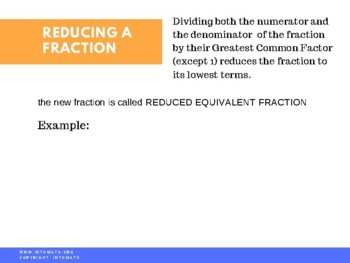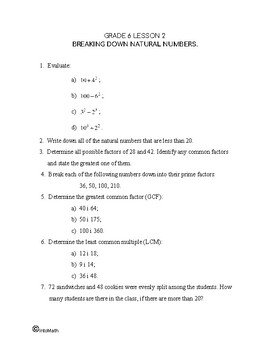CA$2.00 In this lesson you will learn about the concept of a fraction and comparing fractions with unlike denominators. A fraction is a part of a whole. A fraction has two components. The number on the top of the line is called the numerator. It tells how many equal parts of the whole are given. The number at the bottom of the fraction line (bar) is called the denominator. The denominator represents the total number of parts that make up a whole. You will practice how to compare fractions with different denominators and why, in order to do that, you need to bring them to the Lowest Common Denominator (LCD). To find the LCD you would need to find a number that is evenly divisible by the denominators of the fractions you are comparing. But remember, when the denominator changes, the numerator changes with it.Grade 6 Workbook (16 pages with answers) CA$4.00

For example, how could you determine which one is greater, 3/4 or 2/5? In order to compare them, express both fractions in terms of the same number of parts that make up a whole.

The Lowest Common Multiple for 4 and 5 is 20. So, the Lowest Common Denominator is also 20. Now, express each fraction in terms of the denominator 20, preserving the ratio.

3/4 is equivalent to 15/20.

And 2/5 is equivalent to 8/20.

Now, that the denominators are the same, only compare the numerators. Compare them as natural numbers: 15 is greater than 8.

Thus, 15/20 is greater than 8/20.

Record it as 15/20 > 8/20.

In this lesson you will also discover how to compare fractions without using a number line and how to determine equivalent fractions.